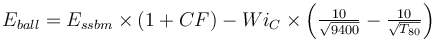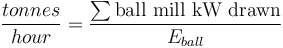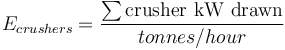# Model:Bond HPGR and ball mill

## HPGR and ball mill circuit

This is a highly experimental model. Use at your own risk!

The high pressure grinding roll (HPGR) and ball mill circuit can be thought of as a variation on the classical single stage ball mill (SSBM) circuit with the final stage of cone crushing replaced by an HPGR. Simulating HPGR has two differences from a classical SSBM circuit with cone crushers: first is that the crusher product tends to be finer (~4 mm versus 9 mm) and the nature of the product is different.

To simulate an HPGR and ball mill circuit, choose the HPGR - BM flowsheet and the Bond/Rowland single stage ball mill model. The model assumes that the circuit is always ball mill limited, and that the crushers have neither volumetric nor power limitations. Engineers (that's you!) must do off-line checks of crusher & HPGR power draw and volumetric limitations to confirm that these assumptions are valid.

### Formulae

The ball mill specific power consumption Eball is determined by deducting a cone crushing component from the Bond/Rowland Essbm specific power consumption. See the base formulae in the Bond SSBM model.$E_{ball} = E_{ssbm} \times (1+CF) - Wi_{C} \times \left ( \tfrac {10}{\sqrt{ 9400 }} - \tfrac {10}{\sqrt{ T_{80} }} \right )$

where CF is the Essbm calibration factor (in the Circuit Settings) for ball mill operating work index versus laboratory ball mill work index. A value of zero means use the Bond/Rowland SSBM formula to predict Eball, a value of -0.05 means use 5% less than Bond/Rowland SSBM formula to predict Eball. CF is a negative number if WiO is less than the laboratory work index.

The circuit throughput is calculated using the ball mill (the circuit is assumed to be ball-limited).$\frac{tonnes}{hour} = \frac{\sum{\text{ball mill kW drawn}} }{E_{ball}}$

The crusher specific power consumption is calculated from the sum of the power drawn in all crushers (which is manually set in the crusher settings) divided by the circuit throughput (which is set by the ball mill circuit). You will need to manually adjust the crusher mechanical efficiency to achieve the Ecrushers observed in the pilot plant. Secondary cone crushers normally consume 50% to 65% of their name-plate power (set the mechanical efficiency to between 0.5 and 0.65). The HPGR mechanical efficiency should be entered such that the Ecrushers indicated equals the sum of the pilot plant EHPGR plus Esec.crushing.$E_{crushers} = \frac{\sum{\text{crusher kW drawn}} }{tonnes/hour}$

## Discussion

The particle size curve of HPGR product is "non-standard" according to Bond's root-2 rule, meaning the transfer size for Bond calculations is "synthetic" (same as for SAG mills). The "microcracking" reported in HPGR product has the same effect as the non-standard particle size distribution, and both of these are simulated together by this two-step procedure:

1. Adjust the overall circuit Essbm calibration factor in the circuit settings to give you the reduction in the ball mill operating work index (WiO) observed in the HPGR testing.
2. Adjust the mechanical efficiency in the HPGR crusher settings to give you the Ecrush observed in the HPGR testing. Allow for the specific power consumption of the secondary crushing stage in the Ecrush, i.e. Ecrush = EHPGR + Esec crush

## Recommended Usage

This is a highly simplified HPGR model that does not consider the volumetric throughput constraints of the crushers. It is suitable for high level comparisons of the likely difference in "energy efficiency" versus SAG milling circuits. It is not intended as a HPGR circuit sizing calculation; factors such as m·dot, pressing pressure and roll speed are not considered.

The throughput of an HPGR plant, once calibrated to a plant or pilot plant test, can be predicted based on drill core Bond testwork. This also allows geometallurgy calculations – drilling an orebody, combined with testing using off-the-shelf Bond test gear (crushing, rod mill & ball mill) can be used for future production forecasting of an HPGR circuit.

## Default Parameter Values

It is necessary to change the SSBM circuit default parameters to simulate an HPGR circuit:

• T80: Crushing circuit product, µm : set to the observed HPGR screen product size (typically 3000 to 5000 µm).
• Essbm calibration factor : set to the reduction in ball mill operation work index observed in the HPGR test (typically -0.05 to -0.10 for a 5% to 10% reduction).
• HPGR crusher model mechanical efficiency : adjust until the Ecrush matches the HPGR test specific energy. Typical number is 0.3 (suggesting 30% energy is consumed in breakage).